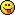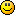## Discuss Scratch

### The shortest FizzBuzz YOU can make!

I attempted to make one in C:
`main(a){for(;a++<100;)if(a%3)if(a%5)printf("%d\n",a);else puts("Buzz");else if(a%5)puts("Fizz");else puts("FizzBuzz");}`
Not exactly the best code golfer…

It also relies on the fact that argc is usually 1 if invoked with no arguments.my latest extension: 2d vector math

### The shortest FizzBuzz YOU can make!

(to bybb) ==0 Can be reduced to <1 for integersLast edited by jokebookservice1 (July 5, 2018 16:46:05)

### The shortest FizzBuzz YOU can make!

jokebookservice1 wrote:

(to bybb) ==0 Can be reduced to <1 for integers```print("".join(((("Fizz"if i%3<1 else"")+("Buzz"if i%5<1 else""))or str(i))+"\n" for i in range(1, 101)))
```
Should be shorted then.

Shorter:
(removed: doesn't work)

Last edited by bybb (July 5, 2018 20:14:24)

Game Over
You'll find me on @LastContinue from now on.

### The shortest FizzBuzz YOU can make!

Batch again
`@echo offset /a i=1:loopset "fb=0"set /a f=%i% %% 3set /a b=%i% %% 5if %f% equ 0 set fb=Fizz0if %b% equ 0 set fb=%fb:~0,-1%Buzz0if %fb% equ 0 set fb=%i%0echo %fb:~0,-1%set /a i=%i%+1if %i% neq 101 goto loop`

Game Over
You'll find me on @LastContinue from now on.

### The shortest FizzBuzz YOU can make!

bybb wrote:

jokebookservice1 wrote:

(to bybb) ==0 Can be reduced to <1 for integers```print("".join(((("Fizz"if i%3<1 else"")+("Buzz"if i%5<1 else""))or str(i))+"\n" for i in range(1, 101)))
```
Should be shorted then.

Shorter:
```print("".join(("Fizz"if i%3<1 else(str(i)if i%5 else"Buzz")+"\n") for i in range(1, 101)))
```
you can still remove a lot of space from that
```print("".join(("Fizz"if i%3<1else(str(i)if i%5else"Buzz")+"\n")for i in range(1,101)))
```
also i seem to be getting the wrong output from this?
`12Fizz4BuzzFizz78FizzBuzz11Fizz1314Fizz1617Fizz19BuzzFizz2223FizzBuzz26Fizz2829Fizz3132Fizz34BuzzFizz3738FizzBuzz41Fizz4344Fizz4647Fizz49BuzzFizz5253FizzBuzz56Fizz5859Fizz6162Fizz64BuzzFizz6768FizzBuzz71Fizz7374Fizz7677Fizz79BuzzFizz8283FizzBuzz86Fizz8889Fizz9192Fizz94BuzzFizz9798FizzBuzz`

rip scratch forums 2007-2017

### The shortest FizzBuzz YOU can make!

xn--cr8h wrote:

bybb wrote:

jokebookservice1 wrote:

(to bybb) ==0 Can be reduced to <1 for integers```print("".join(((("Fizz"if i%3<1 else"")+("Buzz"if i%5<1 else""))or str(i))+"\n" for i in range(1, 101)))
```
Should be shorted then.

Shorter:
```print("".join(("Fizz"if i%3<1 else(str(i)if i%5 else"Buzz")+"\n") for i in range(1, 101)))
```
you can still remove a lot of space from that
```print("".join(("Fizz"if i%3<1else(str(i)if i%5else"Buzz")+"\n")for i in range(1,101)))
```
also i seem to be getting the wrong output from this?
`-snip-`
Ignore, I didn't realise it didn't output “FizzBuzz” on cases of both %3 and %5…

Game Over
You'll find me on @LastContinue from now on.

### The shortest FizzBuzz YOU can make!

Here's a simple (and non-'hacked'-block) Scratch version that I thought'd be a bit of fun to work through (in particular, see challenges at end):

`when [timer v]>(0)delete (all v) of [list v]set [i v] to when [timer v]>(i)add (i) to [list v]change [i v] by (1)when [timer v]>((i)-((i) mod (3)))replace item (last v) of [list v] with [fizz]when [timer v]>((i)-((i) mod (5)))replace item (last v) of [list v] with [buzz]when [timer v]>((i)-((i) mod (15)))replace item (last v) of [list v] with [fizzbuzz]`

It's important to note that above relies on the layering of these timer hat scripts in the editor – the scripts must be ordered as given above, first on lowest layer to last on top layer. (That's in Scratch 2. For Scratch 3 you have to create the scripts in the order given above, and layering in script editor doesn't matter – at least, not at the moment it doesn't…)

One thing of interest here is that I think there's a very slight chance it can ‘randomly’ get an item wrong (though I suspect that's not the case with the current version (v461) of the Scratch player due to one of its particular ‘foibles’).

–So here's a bit of a challenge: who can see how that could happen…?Another challenge… can you see the out-of-bounds list accesses? –And can you see why they just happen to not matter here?
And finally… under what not unusual situation will it (always) go wrong? (How, and why…?)

Last edited by TheLogFather (July 6, 2018 09:46:42)

Siggy the Kumquat slayer:

### The shortest FizzBuzz YOU can make!

I've made Fizzbuzz using Lua, with the ability to easily expand it.

for I = 1, 100 do – Loops through the numbers 1 to 100 using the variable ‘I’
a = I .. “ ” – Makes the output look a bit neater
if I % 3 == 0 then a = a .. “Fizz” end – This is the part that actually does stuff
if I % 5 == 0 then a = a .. “Buzz” end – Copy this and change the ‘5’ and ‘Buzz’ to whatever you want to expand this piece of code
print(a)
end
io.read() – This allows you to see all outputs in the console, you can remove this line if you wish to do so.

Last edited by SuperJumpCube (July 6, 2018 12:06:24)

I am a programmer that decides to sometimes make small things on scratch for the fun of it.

### The shortest FizzBuzz YOU can make!

Here is a “FizzBuzz” program in Perl 5:
```for(1..100)
{
if(\$_%5==0&&\$_%3==0)
{
print"FizzBuzz\n";
}
elsif(\$_%5==0)
{
print"Buzz\n";
}
elsif(\$_%3==0)
{
print"Fizz\n";
}
else
{
print"\$_\n";
}
}
```
That was way too easy…
There are more short-cuts I could have used here.

Edit:
More shortcuts:
```for(1..100)
{
if(\$_%15==0)
{
print"FizzBuzz\n";
}
elsif(\$_%5==0)
{
print"Buzz\n";
}
elsif(\$_%3==0)
{
print"Fizz\n";
}
else
{
print"\$_\n";
}
}
```
There are still many more shortcuts I could use.

Last edited by red_king_cyclops (Aug. 4, 2018 15:19:13)

2+2=4
2*2=4
2^2=4
2^^2=4
2^^^2=4
2^^^^2=4

I see a pattern.

### The shortest FizzBuzz YOU can make!

I apologize for necroposting, but technically the last post of this topic was posted under a month ago.

red_king_cyclops wrote:

Here is a “FizzBuzz” program in Perl 5:
-snip-
There are still many more shortcuts I could use.

More shortcuts:
```for(1..100) {
if(\$_%3==0) {print"Fizz";}
if(\$_%5==0) {print"Buzz";}
elsif(\$_%3!=0) {print"\$_";}
print"\n"; }
```

Now, without any unnecessary whitespace:
```for(1..100){if(\$_%3==0){print"Fizz";}if(\$_%5==0){print"Buzz";}elsif(\$_%3!=0){print"\$_";}print"\n";}
```
That's only 100 bytes!

There are probably more shortcuts out there to use.

```for(my \$count = 1; \$count <= 100; \$count++)
{
if(\$count % 15 == 0)
{
print"FizzBuzz\n";
}
elsif(\$count % 5 == 0)
{
print"Buzz\n";
}
elsif(\$count % 3 == 0)
{
print"Fizz\n";
}
else
{
print"\$count\n";
}
}
```

Last edited by red_king_cyclops (Aug. 5, 2018 11:48:40)

2+2=4
2*2=4
2^2=4
2^^2=4
2^^^2=4
2^^^^2=4

I see a pattern.

### The shortest FizzBuzz YOU can make!

^ Not a necropost since it's relevant to the topic and these sorts of topics can't really “die” just become inactive.

Game Over
You'll find me on @LastContinue from now on.

### The shortest FizzBuzz YOU can make!

bybb wrote:

^ Not a necropost since it's relevant to the topic and these sorts of topics can't really “die” just become inactive.
Thank you for correcting me.

TheMonsterOfTheDeep wrote:

I attempted to make one in C:
`main(a){for(;a++<100;)if(a%3)if(a%5)printf("%d\n",a);else puts("Buzz");else if(a%5)puts("Fizz");else puts("FizzBuzz");}`
Not exactly the best code golfer…

It also relies on the fact that argc is usually 1 if invoked with no arguments.I just want to point out that you forgot the:
```#include <stdio.h>
```

Last edited by red_king_cyclops (Aug. 4, 2018 15:22:15)

2+2=4
2*2=4
2^2=4
2^^2=4
2^^^2=4
2^^^^2=4

I see a pattern.

### The shortest FizzBuzz YOU can make!

red_king_cyclops wrote:

TheMonsterOfTheDeep wrote:

I attempted to make one in C:
`main(a){for(;a++<100;)if(a%3)if(a%5)printf("%d\n",a);else puts("Buzz");else if(a%5)puts("Fizz");else puts("FizzBuzz");}`
Not exactly the best code golfer…

It also relies on the fact that argc is usually 1 if invoked with no arguments.I just want to point out that you forgot the:
```#include <stdio.h>
```
AFAIK, you don't actually need to include stdio on some (?) versions of C. In any case it compiles fine with GCC which was the main point.

my latest extension: 2d vector math

### The shortest FizzBuzz YOU can make!

```def a(n,m):
print(("Fizz"if n%3<1 else"")+("Buzz"if n%5<1 else"")or str(n))
a(n+1,m)if(n<m)else""
```
I wanted to do a short one based on recursion.

Game Over
You'll find me on @LastContinue from now on.

### The shortest FizzBuzz YOU can make!

This Perl code is only 92 bytes long:
```for(1..100){printf"%s%s%s\n",\$_%3==0?"Fizz":"",\$_%5==0?"Buzz":"",\$_%3!=0&&\$_%5!=0?"\$_":"";}
```
Now with whitespace and extra parentheses:
```for(1..100)
{
printf"%s%s%s\n", (\$_ % 3 == 0) ? ("Fizz") : (""), (\$_ % 5 == 0) ? ("Buzz") : (""), ((\$_ % 3 != 0) && (\$_ % 5 != 0)) ? ("\$_") : ("");
}
```
Edit: Only 90 bytes now:
```printf"%s%s%s\n",\$_%3==0?"Fizz":"",\$_%5==0?"Buzz":"",\$_%3!=0&&\$_%5!=0?"\$_":"" for 1..100;
```
Another edit: Only 84 bytes now:
```print \$_%3==0?"Fizz":"",\$_%5==0?"Buzz":"",\$_%3!=0&&\$_%5!=0?"\$_":"","\n" for 1..100;
```

Last edited by red_king_cyclops (Aug. 7, 2018 21:46:59)

2+2=4
2*2=4
2^2=4
2^^2=4
2^^^2=4
2^^^^2=4

I see a pattern.

### The shortest FizzBuzz YOU can make!

Here's my try:
`[print("Fizz"*(i%3<1)+"Buzz"*(i%5<1)or i)for i in range(100)]`

### The shortest FizzBuzz YOU can make!

Does this techincally count as the shortest fizzbuzz for C?
I mean it's the shortest one it just uses a custom library```#include "fizzbuzz.h"
OPEN FIZZBUZZ { OPEN i; SET LOOP { CALC }}
```

fizzbuzz.h
```#include <stdio.h>
#define OPEN int
#define FIZZBUZZ main(void)
#define SET i = 1;
#define LOOP for(; i<=100; ++i)
#define CALC printf("%d %s%s\n", i, i%3?"":"Fizz", i%5?"":"Buzz");
```### The shortest FizzBuzz YOU can make!

Wettining wrote:

Does this techincally count as the shortest fizzbuzz for C?
I mean it's the shortest one it just uses a custom library```#include "fizzbuzz.h"
OPEN FIZZBUZZ { OPEN i; SET LOOP { CALC }}
```

fizzbuzz.h
```#include <stdio.h>
#define OPEN int
#define FIZZBUZZ main(void)
#define SET i = 1;
#define LOOP for(; i<=100; ++i)
#define CALC printf("%d %s%s\n", i, i%3?"":"Fizz", i%5?"":"Buzz");
```
Good code. You gave me an idea…

Here is the shortest fizzbuzz in Python 3:
```import fizzbuzz
fizzbuzz.fizzbuzz()
```
This is a joke.

Last edited by red_king_cyclops (Aug. 7, 2018 21:41:50)

2+2=4
2*2=4
2^2=4
2^^2=4
2^^^2=4
2^^^^2=4

I see a pattern.

### The shortest FizzBuzz YOU can make!

.

Last edited by onlyNones (Sept. 22, 2020 10:37:21)

`########################## E ^ ^       ^                   | | v  v |v|  |||v   | N | | ^     |            ^      v | |  | | v  ||v    v C | ^ |  ^  |            |        | |  | |    ||     v O ^ | |  |  |  ^         |        | |  v |    ||       D | | | ^|  |  |         |        | v    v    ||       E | | ^ ||  |  |         |        v           vv       D ##########################`